Next: Quasi-static processes Up: Heat and work Previous: Macrostates and microstates

The microscopic interpretation of heat and work

Consider a macroscopic systemwhich is known to be in a given macrostate. To be more exact, consider an ensemble of similar macroscopic systems, where each system in the ensemble is in one of the many microstates consistent with the given macrostate. There are two fundamentally different ways in which the average energy ofcan change due to interaction with its surroundings. If the external parameters of the system remain constant then the interaction is termed a purely thermal interaction. Any change in the average energy of the system is attributed to an exchange of heat with its environment. Thus,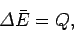(120)

where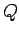is the heat absorbed by the system. On a microscopic level, the energies of the individual microstates are unaffected by the absorption of heat. In fact, it is the distribution of the systems in the ensemble over the various microstates which is modified.

Suppose that the systemis thermally insulated from its environment. This can be achieved by surrounding it by an adiabatic envelope (i.e., an envelope fabricated out of a material which is a poor conductor of heat, such a fiber glass). Incidentally, the term adiabatic is derived from the Greek adiabatos which means impassable.'' In scientific terminology, an adiabatic process is one in which there is no exchange of heat. The systemis still capable of interacting with its environment via its external parameters. This type of interaction is termed mechanical interaction, and any change in the average energy of the system is attributed to work done on it by its surroundings. Thus,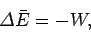(121)

where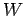is the work done by the system on its environment. On a microscopic level, the energy of the system changes because the energies of the individual microstates are functions of the external parameters [see Eq. (119)]. Thus, if the external parameters are changed then, in general, the energies of all of the systems in the ensemble are modified (since each is in a specific microstate). Such a modification usually gives rise to a redistribution of the systems in the ensemble over the accessible microstates (without any heat exchange with the environment). Clearly, from a microscopic viewpoint, performing work on a macroscopic system is quite a complicated process. Nevertheless, macroscopic work is a quantity which can be readily measured experimentally. For instance, if the systemexerts a force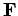on its immediate surroundings, and the change in external parameters corresponds to a displacement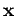of the center of mass of the system, then the work done byon its surroundings is simply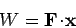(122)

i.e., the product of the force and the displacement along the line of action of the force. In a general interaction of the systemwith its environment there is both heat exchange and work performed. We can write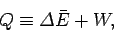(123)

which serves as the general definition of the absorbed heat(hence, the equivalence sign). The quantityis simply the change in the mean energy of the system which is not due to the modification of the external parameters. Note that the notion of a quantity of heat has no independent meaning apart from Eq. (123). The mean energy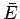and work performedare both physical quantities which can be determined experimentally, whereasis merely a derived quantity.Next: Quasi-static processes Up: Heat and work Previous: Macrostates and microstates
Richard Fitzpatrick 2006-02-02